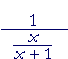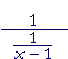S k i l l
i n
A L G E B R A

Complex fractions -- Division

Back to Section 1

 A complex fraction looks like this:The numerator and/or the denominator are themselves fractions.

To simplify a complex fraction, we can immediately apply the definition of division (Lesson 5):

 ab =  a· 1b

Any fraction is equal to the numerator times the reciprocal
of the denominator.

Therefore,= pq · nm

Problem 1.   State in words how to simplify a complex fraction.

Rewrite it as the numerator times the reciprocal
of the denominator.

 Example 1.   SimplifySolution.= x2 − 25   x8 · x³ x − 5
 = (x + 5)(x − 5)        x8 · x³ x − 5 = x + 5   x5

Division -- which effectively this is -- becomes multiplication by the reciprocal.on canceling the x + 2's.

Problem 2.   Simplify.

 a)= 6 x5 · x28 = 3  4x3
 b)= 4   x − 1 · 1   x − 1 = _4_      x2 − 2x + 1
 c)= (x + 2) · x − 2x + 2 = x − 2
 d)= x + 2x + 1 · x − 2x − 1 = x2 − 4x2 − 1
 e)= −h    x(x + h) · 1h = − 1     x(x + h)

The h's cancel.  And according to the Rule of Signs, the product is negative.  (It's all right to leave the product in its factored form.)

 f)= (x + 2)(x − 2)       3x2 · _x_       (x + 2)(x + 3)
 = x − 2  3x · 1   x + 3 = x − 2   3x(x + 3)

Example 3.  If a complex fraction looks like this ---- then we can simplify it by multiplying the numerator and denominator by c.Problem 3.   Simplify the following.= _(x + 1) − 1_(x + 1)(x − 1) = __x__x2 − 1
 Example 4.   SimplifySolution.    1-over any number is its reciprocal.  Therefore,= 43

Problem 4.   Simplify the following.

 a)= x + 1   x b)= x − 1Next Lesson:  Adding algebraic fractions

Please make a donation to keep TheMathPage online.
Even \$1 will help.